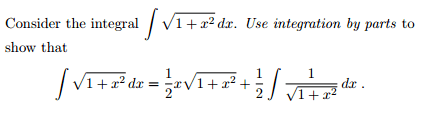# Integration by parts

## Homework Statement

[/B]## Homework Equations

∫ f(x) g'(x) dx = f(x) g(x) - ∫ f '(x) g(x) dx

f(x)=√(1+x^2)
f '(x)=x * 1/√(1+x^2)

g'(x)=1
g(x)=x

## The Attempt at a Solution

∫ √(1+x^2) * 1 dx
=x * √(1+x^2) - ∫ x^2 * 1/√(1+x^2) dx

Further integration just makes the result look further from what it's supposed to look like